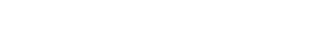# Levenshtein Python Algo implementation Wagner & FischerHere is the levenshtein python implementation of the Wagner & Fischer algorithm (Wagner-Fischer). It allows to calculate the distance of Levenshtein (distance between two strings of characters).

First, the goal of the algorithm is to find the minimum cost. Minimal cost to transform one channel into another. Then a recursive function allows to return the minimum number of transformation. And to transform a substring of A with n characters into a substring of B with the same number of characters. The solution is given. We must calculate the distance between the 2 letters at the same position in the chain A and B. Then Either the letters and the previous letter are identical. Either there is a difference and in this case we calculate 3 costs. So the first is to delete a letter from the chain A. And insert a letter in the chain A to substitute a letter from the chain A. Then we can then find the minimum cost.

Example of levenshtein python implementation :

```import numpy as np

def levenshtein(chaine1, chaine2):
taille_chaine1 = len(chaine1) + 1
taille_chaine2 = len(chaine2) + 1
levenshtein_matrix = np.zeros ((taille_chaine1, taille_chaine2))
for x in range(taille_chaine1):
levenshtein_matrix [x, 0] = x
for y in range(taille_chaine2):
levenshtein_matrix [0, y] = y

for x in range(1, taille_chaine1):
for y in range(1, taille_chaine2):
if chaine1[x-1] == chaine2[y-1]:
levenshtein_matrix [x,y] = min(
levenshtein_matrix[x-1, y] + 1,
levenshtein_matrix[x-1, y-1],
levenshtein_matrix[x, y-1] + 1
)
else:
levenshtein_matrix [x,y] = min(
levenshtein_matrix[x-1,y] + 1,
levenshtein_matrix[x-1,y-1] + 1,
levenshtein_matrix[x,y-1] + 1
)
return (levenshtein_matrix[taille_chaine1 - 1, taille_chaine2 - 1])

print("distance de levenshtein = " + str(levenshtein("Lorem ipsum dolor sit amet", "Laram zpsam dilir siy amot")))```

To calculate the Levenshtein distance with a non-recursive algorithm. We use a matrix which contains the Levenshtein distances. Then These are the distances between all the prefixes of the first string and all the prefixes of the second string.

Also we can dynamically calculate the values ​​in this matrix. The last calculated value will be the Levenshtein distance between the two whole chains.

Finally there are many use cases of the Levenshtein distance. Levenshtein distance is used in domains. Computational linguistics, molecular biology. And again bioinformatics, machine learning. Also deep learning, DNA analysis. In addition, a program that does spell checking uses, for example, the Levenshtein distance.

## Links on the Wagner & Fischer algorithm (Wagner-Fischer) and the Levenshtein python distance or other language:

https://graal.hypotheses.org/tag/algorithme-de-wagner-fischer

https://fr.wikipedia.org/wiki/Algorithme_de_Wagner-Fischer

https://fr.wikipedia.org/wiki/Distance_de_Levenshtein

https://medium.com/@sddkal/wagner-fischer-algorithm-be0d96893f6d

https://www.python-course.eu/levenshtein_distance.php

## Internal links on algorithms

https://128mots.com/index.php/category/python/

https://128mots.com/index.php/category/graphes/